Ex 7.2

Chapter 7 Class 8 Comparing Quantities
Serial order wise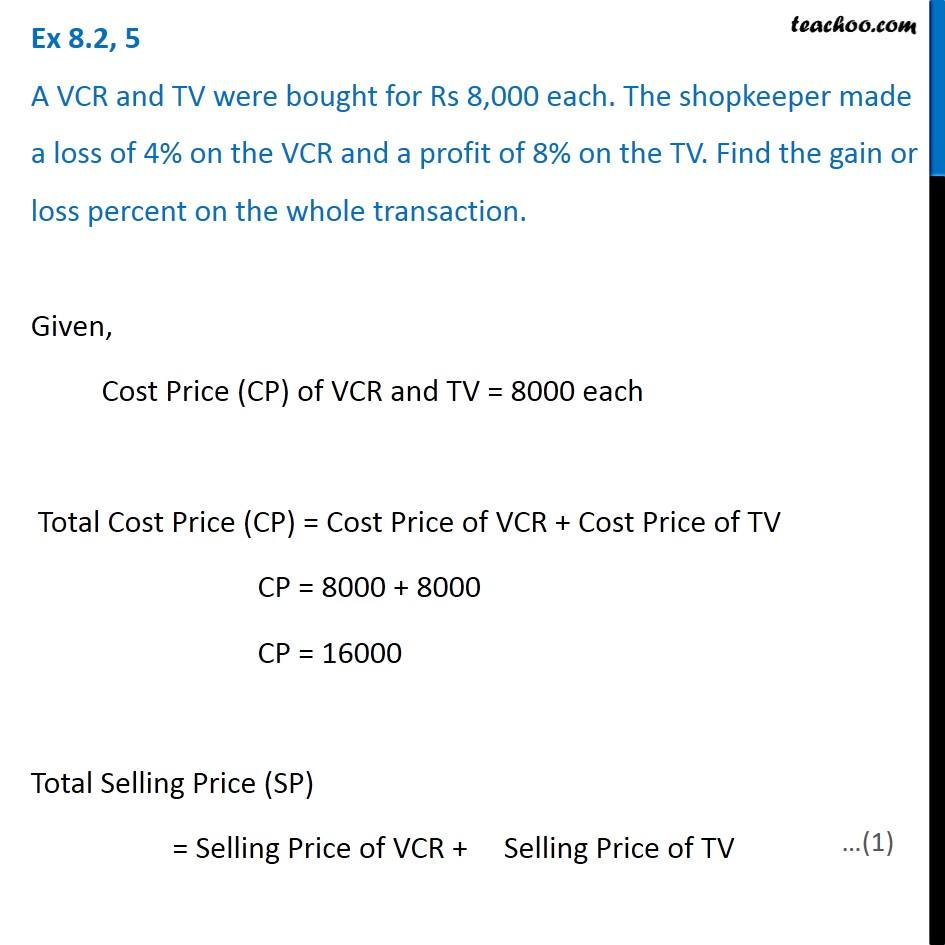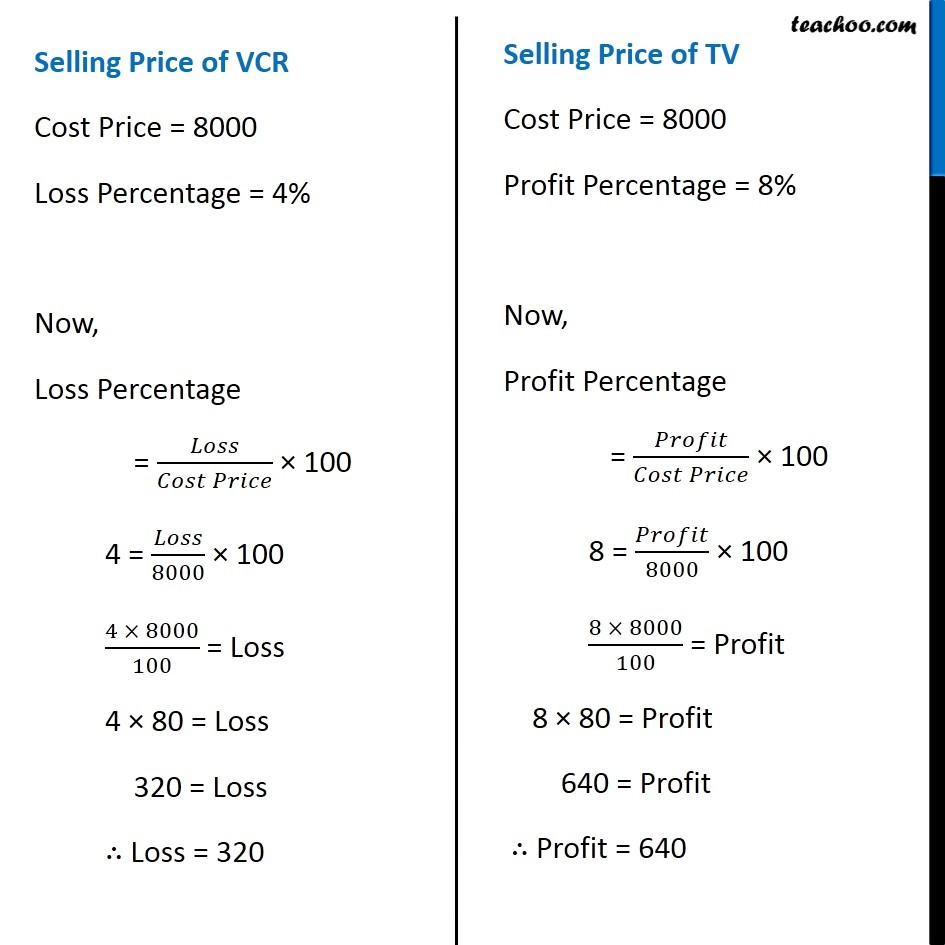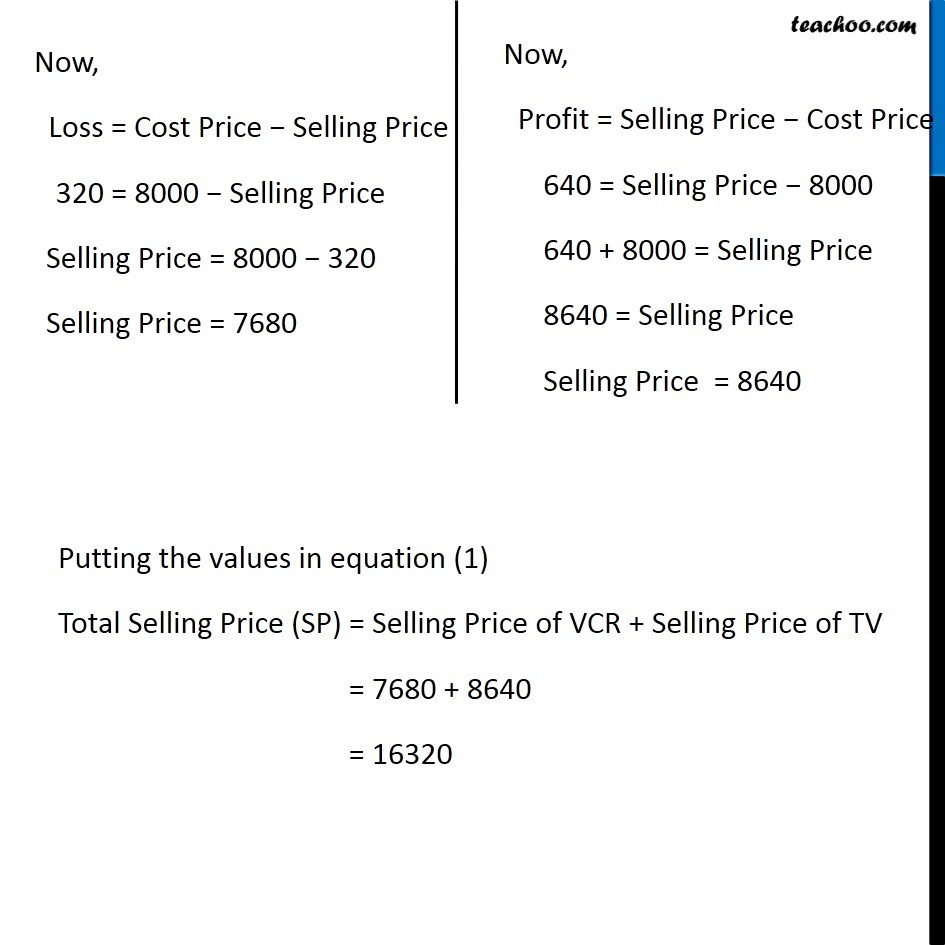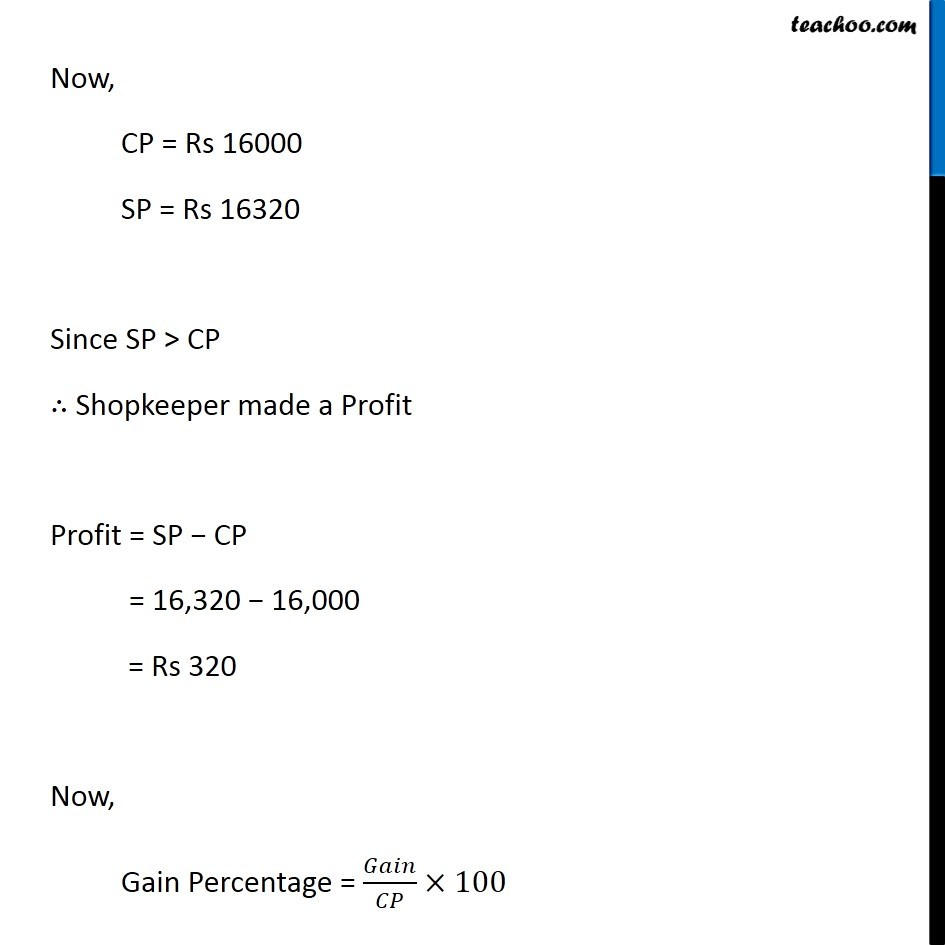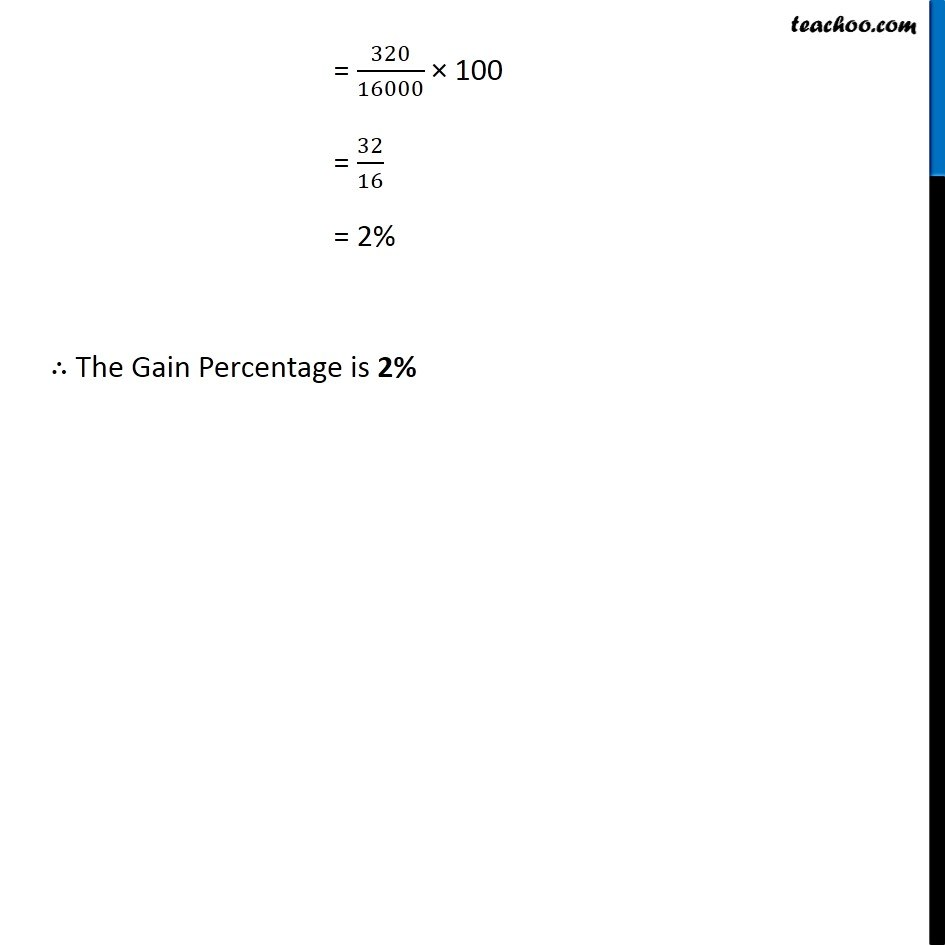Learn in your speed, with individual attention - Teachoo Maths 1-on-1 Class

### Transcript

Question 5 A VCR and TV were bought for Rs 8,000 each. The shopkeeper made a loss of 4% on the VCR and a profit of 8% on the TV. Find the gain or loss percent on the whole transaction. Given, Cost Price (CP) of VCR and TV = 8000 each Total Cost Price (CP) = Cost Price of VCR + Cost Price of TV CP = 8000 + 8000 CP = 16000 Total Selling Price (SP) = Selling Price of VCR + Selling Price of TV Selling Price of VCR Cost Price = 8000 Loss Percentage = 4% Now, Loss Percentage = 𝐿𝑜𝑠𝑠/(𝐶𝑜𝑠𝑡 𝑃𝑟𝑖𝑐𝑒) × 100 4 = 𝐿𝑜𝑠𝑠/8000 × 100 (4 × 8000)/100 = Loss 4 × 80 = Loss 320 = Loss ∴ Loss = 320 Selling Price of TV Cost Price = 8000 Profit Percentage = 8% Now, Profit Percentage = 𝑃𝑟𝑜𝑓𝑖𝑡/(𝐶𝑜𝑠𝑡 𝑃𝑟𝑖𝑐𝑒) × 100 8 = 𝑃𝑟𝑜𝑓𝑖𝑡/8000 × 100 (8 × 8000)/100 = Profit 8 × 80 = Profit 640 = Profit ∴ Profit = 640 Now, Loss = Cost Price − Selling Price 320 = 8000 − Selling Price Selling Price = 8000 − 320 Selling Price = 7680 Now, Profit = Selling Price − Cost Price 640 = Selling Price − 8000 640 + 8000 = Selling Price 8640 = Selling Price Selling Price = 8640 Putting the values in equation (1) Total Selling Price (SP) = Selling Price of VCR + Selling Price of TV = 7680 + 8640 = 16320 Now, CP = Rs 16000 SP = Rs 16320 Since SP > CP ∴ Shopkeeper made a Profit Profit = SP − CP = 16,320 − 16,000 = Rs 320 Now, Gain Percentage = 𝐺𝑎𝑖𝑛/𝐶𝑃×100 = 320/16000 × 100 = 32/16 = 2% ∴ The Gain Percentage is 2%